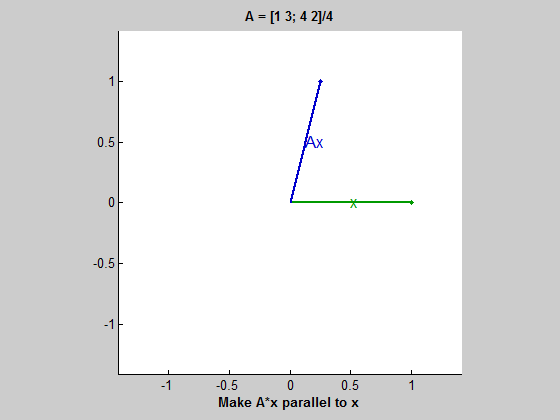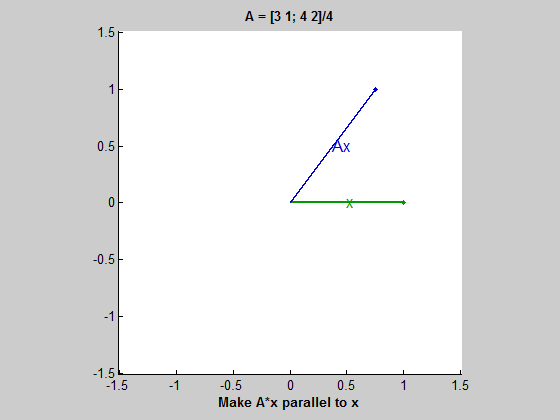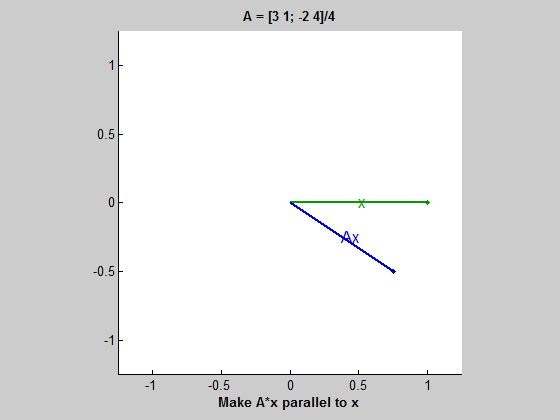eigshow, week 1

One of my all-time favorite MATLAB examples is eigshow.Contents

eigshow

The eigshow example has been part of MATLAB for a long time and has always been one of my favorites.

When you start eigshow, a 2-by-2 matrix is shown in the title bar. There is also a green vector x and a blue vector Ax, the image of x under the mapping induced by A. The default values, shown in the animated gif above, are

format rat, format compact
A = [1 3; 4 2]/4
x = [1; 0]
Ax = A*x
A =
1/4            3/4
1              1/2
x =
1
0
Ax =
1/4
1

Ellipse

Now move x with the mouse. x stays on the unit circle. Ax goes wherever A takes x, generating the blue curve, an ellipse.

Line 'em up

Run eigshow yourself. Don't just watch the animation. The instructions at the bottom of the screen say " Make A*x parallel to x ". When you do this -- when the blue and green vectors are pointing in the same direction -- you have found an eigenvector. The green vector is the eigenvector and the length of the overlapping blue vector is the eigenvalue.

Eigenvectors

One eigenvector of this particular matrix is in the first or third quadrant, making an angle of roughly 60 degrees with the horizontal axis. The blue vector is longer than the green. The eigenvalue is actually 5/4.

The other eigenvector is obtained when the blue vector is pointing in the opposite direction from the green, because the eigenvalue is negative. The eigenvector is in the second or fourth quadrant, at an angle of about 45 degrees. The eigenvalue is -1/2.

Notice that the eigenvectors are not the major and minor axes of the ellipse. This matrix is not quite symmetric. If it were symmetric, the eigenvectors would be the axes.

A Second Matrix

The title bar of eigshow is a pull-down menu that offers a dozen different matrices. Our second example swaps the top two elements of the first example.

A = [3 1; 4 2]/4
A =
3/4            1/4
1              1/2Now the aspect ratio of the ellipse is quite a bit larger. Both eigenvalues are positive. If you watch carefully, you can see that Ax goes around in the same direction as x. Our first matrix has one negative eigenvalue and so x and Ax travel in opposite directions.

A Third Matrix

Now choose the matrix that swaps the bottom two elements and flips one sign.

A = [3 1; -2 4]/4
A =
3/4            1/4
-1/2            1Trying to make A*x parallel to x is frustrating. You can't do it. More precisely, you would have to come off the page or screen to do it. This matrix does not have any real eigenvalues and eigenvectors. Its eigenvalues and eigenvectors are complex.

More later

I plan to do more examples with eigshow in my next blog posting.

Published with MATLAB® R2013b

|